# You are evaluating two different silicon wafer milling machines. The Techron I costs \$258,000, has a three-year life, and has pretax operating costs of \$69,000 per year. The Techron II costs \$450,000, has a five-year life, and has pretax operating costs of \$42,000 per year. For both milling machines, use straight-line depreciation to zero over the project’s life and assume a salvage value of \$46,000. If your tax rate is 25 percent and your discount rate is 10 percent, compute the EAC for both machines. (A negative answer should be indicated by a minus sign. Do not round intermediate calculations and round your answers to 2 decimal places, e.g., 32.16.)

Question
21 views
 You are evaluating two different silicon wafer milling machines. The Techron I costs \$258,000, has a three-year life, and has pretax operating costs of \$69,000 per year. The Techron II costs \$450,000, has a five-year life, and has pretax operating costs of \$42,000 per year. For both milling machines, use straight-line depreciation to zero over the project’s life and assume a salvage value of \$46,000. If your tax rate is 25 percent and your discount rate is 10 percent, compute the EAC for both machines. (A negative answer should be indicated by a minus sign. Do not round intermediate calculations and round your answers to 2 decimal places, e.g., 32.16.)

check_circle

Step 1

Equivalent Annual cost (EAC) is the total cost incurred by the company annually for operating and maintaining an asset within that company.

Net present value is the present value of the future cash flows at a specified time period and discount rate. Discount rate is the minimum rate of lending which is also known as cost of capital.

Given Information: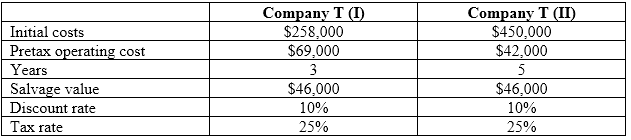Step 2

Formulae used: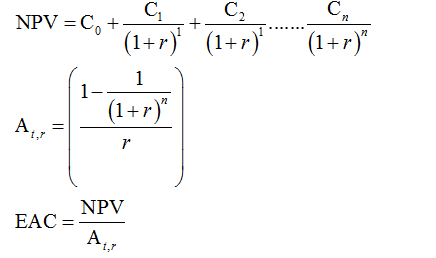Step 3

Calculations for Company T (I):

Firstly, calculate the depreciation by suing straight line method: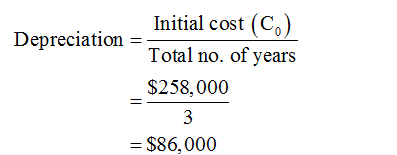To calculate the NPV, net cash flows are required which are calculated as follows: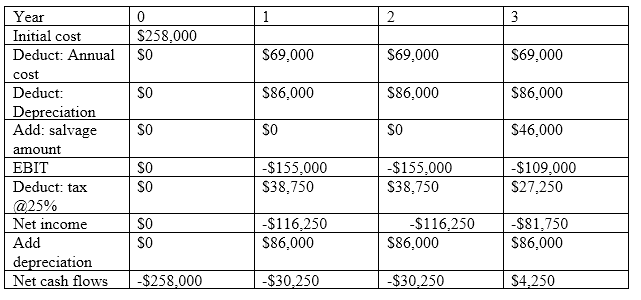By using excel sheet or above formula, the NPV can be calculated which is as follows: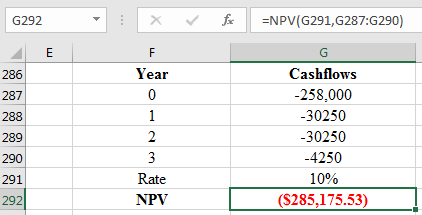By using above formula calculations for the PVIFA@10% for three years and EAC are as follows: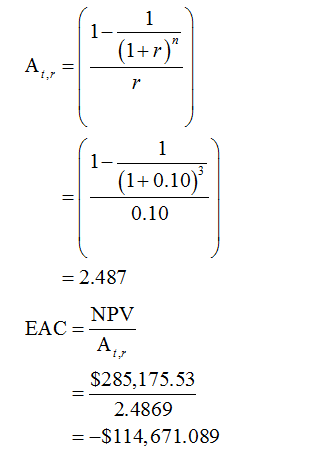...

### Want to see the full answer?

See Solution

#### Want to see this answer and more?

Solutions are written by subject experts who are available 24/7. Questions are typically answered within 1 hour.*

See Solution
*Response times may vary by subject and question.
Tagged in